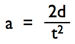# Gravitational Acceleration

We all understand that if we hold something up in the air and then let go, it will fall to the ground. Things fall because of gravity. Gravity is an attractive force between all things that have mass*.  It is one of the fundamental forces of nature. Gravity causes objects with mass to accelerate towards each other. The rate of acceleration depends on the mass of the objects and their proximity. The more mass an object contains, the more it will attract other objects. The closer an object is to another the greater the attraction between them will be.

Near the earths surface, acceleration due to gravity is 9.8 m/s2.  This means that an object, such as a ball, dropped from a small distance above the ground will accelerate towards the ground at 9.8 m/s2. If the ball starts with a velocity of zero, it will be traveling at 9.8 m/s after falling for one second. After two seconds it will be traveling twice that fast (19.6 m/s). After 10 seconds it will be moving at 98 m/s. if air resistance can be ignored, it will continue accelerating until some other force (such as resistance from the ground) intervenes. Understanding the relationship between velocity, acceleration and distance traveled allows us to calculate the velocity and position of a moving at any time as long as its rate of acceleration is known.

I simple cases were a objects initial velocity is zero, the relationship between acceleration (a), distance traveled (d) and time (t) isUsing the relationship it is possible to calculate the force of gravity on an object if the time it takes to to fall a set distance is known*.

The following interactive illustration explores the relationship between distance traveled and velocity of objects under different rates of constant acceleration due to gravity. Below the illustration is a youtube video demonstrating its use. Be sure to also take a look at the time distance and acceleration practice problems to test your understanding of the concepts covered by this illustration.

Demonstration video:

*Assuming air resistance can be ignored and the object has an initial velocity of 0.
Subject tag: